## Damn Cool Algorithms: Spatial indexing with Quadtrees and Hilbert Curves

Last Thursday night at Oredev, after the sessions, was "Birds of a Feather" - a sort of mini-unconference. Anyone could write up a topic on the whiteboard; interested individuals added their names, and each group got allocated a room to chat about the topic. I joined the "Spatial Indexing" group, and we spent a fascinating hour and a half talking about spatial indexing methods, reminding me of several interesting algorithms and techniques.

Spatial indexing is increasingly important as more and more data and applications are geospatially-enabled. Efficiently querying geospatial data, however, is a considerable challenge: because the data is two-dimensional (or sometimes, more), you can't use standard indexing techniques to query on position. Spatial indexes solve this through a variety of techniques. In this post, we'll cover several - quadtrees, geohashes (not to be confused with geohashing), and space-filling curves - and reveal how they're all interrelated.

Quadtrees are a very straightforward spatial indexing technique. In a Quadtree, each node represents a bounding box covering some part of the space being indexed, with the root node covering the entire area. Each node is either a leaf node - in which case it contains one or more indexed points, and no children, or it is an internal node, in which case it has exactly four children, one for each quadrant obtained by dividing the area covered in half along both axes - hence the name.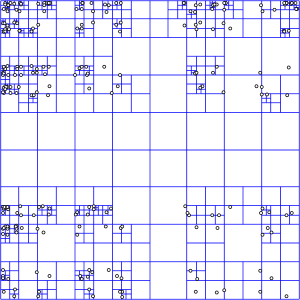A representation of how a quadtree divides an indexed area. Source: Wikipedia

Inserting data into a quadtree is simple: Starting at the root, determine which quadrant your point occupies. Recurse to that node and repeat, until you find a leaf node. Then, add your point to that node's list of points. If the list exceeds some pre-determined maximum number of elements, split the node, and move the points into the correct subnodes.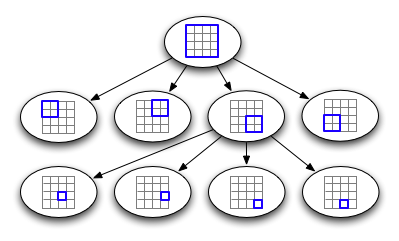A representation of how a quadtree is structured internally.

To query a quadtree, starting at the root, examine each child node, and check if it intersects the area being queried for. If it does, recurse into that child node. Whenever you encounter a leaf node, examine each entry to see if it intersects with the query area, and return it if it does.

Note that a quadtree is very regular - it is, in fact, a trie, since the values of the tree nodes do not depend on the data being inserted. A consequence of this is that we can uniquely number our nodes in a straightforward manner: Simply number each quadrant in binary (00 for the top left, 10 for the top right, and so forth), and the number for a node is the concatenation of the quadrant numbers for each of its ancestors, starting at the root. Using this system, the bottom right node in the sample image would be numbered 11 01.

If we define a maximum depth for our tree, then, we can calculate a point's node number without reference to the tree - simply normalize the node's coordinates to an appropriate integer range (for example, 32 bits each), and then interleave the bits from the x and y coordinates -each pair of bits specifies a quadrant in the hypothetical quadtree.

### Geohashes

This system might seem familiar: it's a geohash! At this point, you can actually throw out the quadtree itself - the node number, or geohash, contains all the information we need about its location in the tree. Each leaf node in a full-height tree is a complete geohash, and each internal node is represented by the range from its smallest leaf node to its largest one. Thus, you can efficiently locate all the points under any internal node by indexing on the geohash by performing a query for everything within the numeric range covered by the desired node.

Querying once we've thrown away the tree itself becomes a little more complex. Instead of refining our search set recursively, we need to construct a search set ahead of time. First, find the smallest prefix (or quadtree node) that completely covers the query area. In the worst case, this may be substantially larger than the actual query area - for example, a small shape in the center of the indexed area that intersects all four quadrants would require selecting the root node for this step.

The aim, now, is to construct a set of prefixes that completely covers the query region, while including as little area outside the region as possible. If we had no other constraints, we could simply select the set of leaf nodes that intersect the query area - but that would result in a lot of queries. Another constraint, then, is that we want to minimise the number of distinct ranges we have to query for.

One approach to doing this is to start by setting a maximum number of ranges we're willing to have. Construct a set of ranges, initially populated with the prefix we identified earlier. Pick the node in the set that can be subdivided without exceeding the maximum range count and will remove the most unwanted area from the query region. Repeat this until there are no ranges in the set that can be further subdivided. Finally, examine the resulting set, and join any adjacent ranges, if possible. The diagram below demonstrates how this works for a query on a circular area with a limit of 5 query ranges.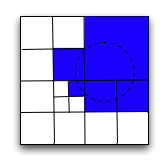How a query for a region is broken into a series of geohash prefixes/ranges.

This approach works well, and it allows us to avoid the need to do recursive lookups - the set of range lookups we do execute can all be done in parallel. Since each lookup can be expected to require a disk seek, parallelizing our queries allows us to substantially cut down the time required to return the results.

Still, we can do better. You may notice that all the areas we need to query in the above diagram are adjacent, yet we can only merge two of them (the two in the bottom right of the selected area) into a single range query, requiring us to do 4 separate queries. This is due in part to the order that our geohashing approach 'visits' subregions, working left to right, then top to bottom in each quad. The discontinuity as we go from top right to bottom left quad results in us having to split up some ranges that we could otherwise make contiguous. If we were to visit regions in a different order, perhaps we could minimise or eliminate these discontinuities, resulting in more areas that can be treated as adjacent and fetched with a single query. With an improvement in efficiency like that, we could do fewer queries for the same area covered, or conversely, the same number of queries, but including less extraneous area.Illustrates the order in which the geohashing approach 'visits' each quad.

### Hilbert Curves

Suppose instead, we visit regions in a 'U' shape. Within each quad, of course, we also visit subquads in the same 'U' shape, but aligned so as to match up with neighbouring quads. If we organise the orientation of these 'U's correctly, we can completely eliminate any discontinuities, and visit the entire area at whatever resolution we choose continuously, fully exploring each region before moving on to the next. Not only does this eliminate discontinuities, but it also improves the overall locality. The pattern we get if we do this may look familiar - it's a Hilbert Curve.

Hilbert Curves are part of a class of one-dimensional fractals known as space-filling curves, so named because they are one dimensional lines that nevertheless fill all available space in a fixed area. They're fairly well known, in part thanks to XKCD's use of them for a map of the internet. As you can see, they're also of use for spatial indexing, since they exhibit exactly the locality and continuity required. For example, if we take another look at the example we used for finding the set of queries required to encompass a circle above, we find that we can reduce the number of queries by one: the small region in the lower left is now contiguous with the region to its right, and whilst the two regions at the bottom are no longer contiguous with each other, the rightmost one is now contiguous with the large area in the upper right.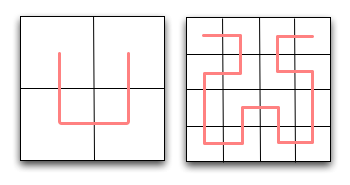Illustrates the order in which a hilbert curve 'visits' each quad.

One thing that our elegant new system is lacking, so far, is a way of converting between a pair of (x,y) coordinates and the corresponding position in the hilbert curve. With geohashing it was easy and obvious - just interleave the x and y coordinates - but there's no obvious way to modify that for a hilbert curve. Searching the internet, you're likely to come across many descriptions of how hilbert curves are drawn, but few if any descriptions of how to find the position of an arbitrary point. To figure this out, we need to take a closer look at how the hilbert cure can be recursively constructed.

The first thing to observe is that although most references to hilbert curves focus on how to draw the curve, this is a distraction from the essential property of the curve, and its importance to us: It's an ordering for points on a plane. If we express a hilbert curve in terms of this ordering, drawing the curve itself becomes trivial - simply a matter of connecting the dots. Forget about how to connect adjacent sub-curves, and instead focus on how we can recursively enumerate the points.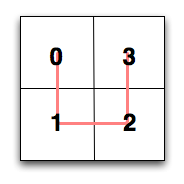Hilbert curves are all about ordering a set of points on a 2d plane

At the root level, enumerating the points is simple: Pick a direction and a start point, and proceed around the four quadrants, numbering them 0 to 3. The difficulty is introduced when we want to determine the order we visit the sub-quadrants in while maintaining the overall adjacency property. Examination reveals that each of the sub-quadrants' curves is a simple transformation of the original curve: there are only four possible transformations. Naturally, this applies recursively to sub-sub quadrants, and so forth. The curve we use for a given quadrant is determined by the curve we used for the square it's in, and the quadrant's position. With a little work, we can construct a table that encapsulates this: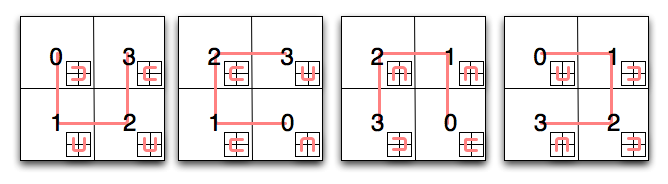Suppose we want to use this table to determine the position of a point on a third-level hilbert curve. For the sake of this example, assume our point has coordinates (5,2) Starting with the first square on the diagram, find the quadrant your point is in - in this case, it's the upper right quadrant. The first part of our hilbert curve position, then, is 3 (11 in binary). Next, we consult the square shown in the inset of square 3 - in this case, it's the second square. Repeat the process: which sub-quadrant does our point fall into? Here, it's the lower left one, meaning the next part of our position is 1, and the square we should consult next is the second one again. Repeating the process one final time, we find our point falls in the upper right sub-sub-quadrant, our final coordinate is 3 (11 in binary). Stringing them together, we now know the position of our point on the curve is 110111 binary, or 55.

Let's be a little more methodical, and write methods to convert between x,y coordinates and hilbert curve positions. First, we need to express our diagram above in terms a computer can understand:

```hilbert_map = { 'a': {(0, 0): (0, 'd'), (0, 1): (1, 'a'), (1, 0): (3, 'b'), (1, 1): (2, 'a')}, 'b': {(0, 0): (2, 'b'), (0, 1): (1, 'b'), (1, 0): (3, 'a'), (1, 1): (0, 'c')}, 'c': {(0, 0): (2, 'c'), (0, 1): (3, 'd'), (1, 0): (1, 'c'), (1, 1): (0, 'b')}, 'd': {(0, 0): (0, 'a'), (0, 1): (3, 'c'), (1, 0): (1, 'd'), (1, 1): (2, 'd')}, }```

In the snippet above, each element of 'hilbert_map' corresponds to one of the four squares in the diagram above. To make things easier to follow, I've identified each one with a letter - 'a' is the first square, 'b' the second, and so forth. The value for each square is a dict, mapping x and y coordinates for the (sub-)quadrant to the position along the line (the first part of the value tuple) and the square to use next (the second part of the value tuple). Here's how we can use this to translate x and y coordinates into a hilbert curve position:

```def point_to_hilbert(x, y, order=16): current_square = 'a' position = 0 for i in range(order - 1, -1, -1): position <<= 2 quad_x = 1 if x & (1 << i) else 0 quad_y = 1 if y & (1 << i) else 0 quad_position, current_square = hilbert_map[current_square][(quad_x, quad_y)] position |= quad_position return position```

The input to this function is the integer x and y coordinates, and the order of the curve. An order 1 curve fills a 2x2 grid, an order 2 curve fills a 4x4 grid, and so forth. Our x and y coordinates, then, should be normalized to a range of 0 to 2order-1. The function works by stepping over each bit of the x and y coordinates, starting with the most significant. For each, it determines which (sub-)quadrant the coordinate lies in, by testing the corresponding bit, then fetches the position along the line and the next square to use from the table we defined earlier. The curve position is set as the least significant 2 bits on the position variable, and at the beginning of the next loop, it's left-shifted to make room for the next set of coordinates.

Let's check that we've written the function correctly by running our example from above through it:

```>>> point_to_hilbert(5,2,3) 55```

Presto! For a further test, we can use the function to generate a complete list of ordered points for a hilbert curve, then use a spreadsheet to graph them and see if we get a hilbert curve. Enter the following expression into an interactive Python interpreter:

```>>> points = [(x, y) for x in range(8) for y in range(8)] >>> sorted_points = sorted(points, key=lambda k: point_to_hilbert(k, k, 3)) >>> print '\n'.join('%s,%s' % x for x in sorted_points) ```

Take the resulting text, paste it into a file called 'hilbert.csv', open it in your favorite spreadsheet, and instruct it to generate a scatter plot. The result is, of course, a nicely plotted hilbert curve!

The inverse of point_to_hilbert is a straightforward reversal of the hilbert_map; implementing it is left as an exercise for the reader.

### Conclusion

There you have it - spatial indexing, from quadtrees to geohashes to hilbert curves. One final observation: If you express the ordered sequence of x,y coordinates required to draw a hilbert curve in binary, do you notice anything interesting about the ordering? Does it remind you of anything?

Just to wrap up, a caveat: All of the indexing methods I've described today are only well-suited to indexing points. If you want to index lines, polylines, or polygons, you're probably out of luck with these methods - and so far as I'm aware, the only known algorithm for effectively indexing shapes is the R-tree, an entirely different and more complex beast.